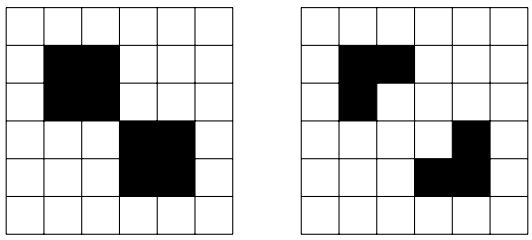시간 제한메모리 제한제출정답맞힌 사람정답 비율
2 초 512 MB0000.000%

## 문제

Conway’s game of life is played on a torus formed of m × n square cells. An m × n torus is just an m × n rectangle where the left and right edges of the rectangle have been glued together, as well as the top and bottom edges. The cells are identified by their coordinates (r, c), indicating the row number and column number. Every cell is either dead or alive. The state of the game is the pattern of live and dead cells on the torus.

Every cell on the torus has exactly 8 neighbors, which are the cells that are horizontally, vertically and diagonally adjacent. In particular, the neighbors of the cells (1, 1) are (0, 0), (0, 1), (0, 2), (1, 0), (1, 2), (2, 0), (2, 1), and (2, 2). The neighbors of the cell (0, 1) are (m, 0), (m, 1), (m, 2), (0, 0), (0, 2), (2, 0), (2, 1), and (2, 2).

At each step in time, the state of the game changes. The state of each cell at step i + 1 is determined from the state of its 8 neighbors at step i, according to the following rules:

• If 1 or less of the neighbors of the cell were alive at step i, then the cell is dead at step i + 1.
• If 2 of the neighbors of the cell were alive at step i, then the state of the cell at step i + 1 is the same as its state at step i.
• If 3 of the neighbors of the cell were alive at step i, then the cell is alive at step i + 1.
• If 4 or more of the neighbors of the cell were alive at step i, then the cell is dead at step i + 1.

The initial state is called the seed of the system. For k ∈ N, we say that a state of the game has period k if, when the game is at this state, then we get back at exactly the same state k steps later. For instance, the configuration illustrated in the left diagram of Figure 2 has period 2: after one step, we obtain the configuration illustrated in the right diagram, and after one step we obtain again the configuration illustrated in the left diagram.

For a given seed, the period of this seed is the smallest k ∈ N such that the game, starting at this seed, eventually reaches a state with period k. Given a seed, your goal is to compute the period of the system.

## 입력

The input consists of several test cases. The first line consists of an integer indicating the number of test cases. Each test case follows. The first line of a test case consists of two positive integers 3 ≤ m ≤ 8 and 3 ≤ n ≤ 8 separated by a single space: m indicates the number of rows of the torus, and n indicates the number of columns. The next m lines represent the seed: each line describes a row and consists of precisely n characters which are either ’x’ for a dead cell or ’o’ for a live cell.

## 출력

For each test case in the input, your program should produce one line. The contents of this line should be a positive integer p > 0 which is the period of the given seed. There should be no blank lines in your output.

## 예제 입력 1

2
3 4
xxxx
xoxx
xxxx
6 6
xxxxxx
xooxxx
xooxxx
xxxoox
xxxoox
xxxxxx


## 예제 출력 1

1
2


## 힌트Figure 2: Illustration of the second input from the Sample Input: a seed with period two, and the configuration to which it leads